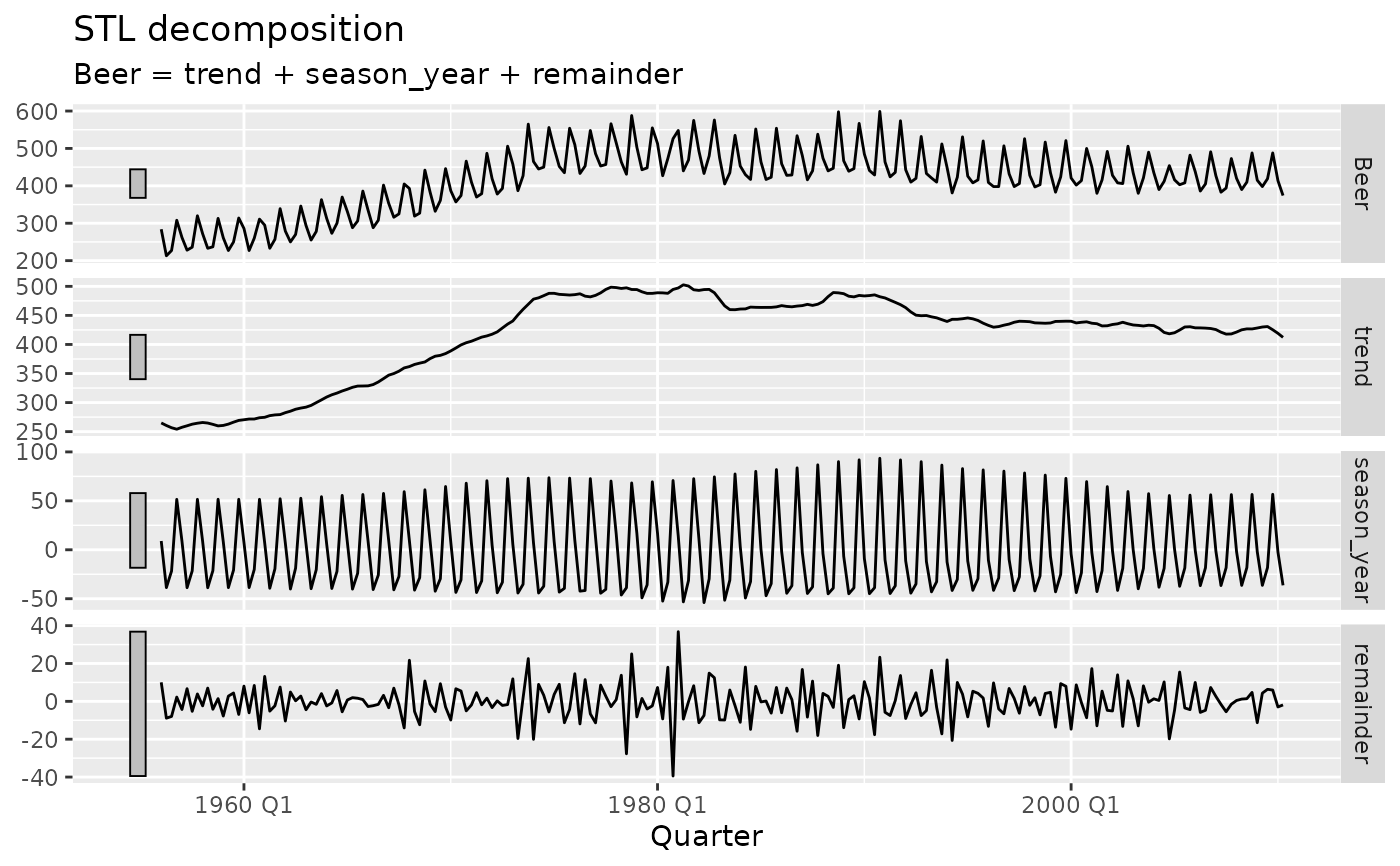Produces a faceted plot of the components used to build the response variable of the dable. Useful for visualising how the components contribute in a decomposition or model.

# S3 method for dcmp_ts
autoplot(object, .vars = NULL, scale_bars = TRUE, level = c(80, 95), ...)

## Arguments

object A dable. The column of the dable used to plot. By default, this will be the response variable of the decomposition. If TRUE, each facet will include a scale bar which represents the same units across each facet. If the decomposition contains distributions, which levels should be used to display intervals? Further arguments passed to ggplot2::geom_line(), which can be used to specify fixed aesthetics such as colour = "red" or size = 3.

## Examples

library(feasts)
library(tsibbledata)
aus_production %>%
model(STL(Beer)) %>%
components() %>%
autoplot()